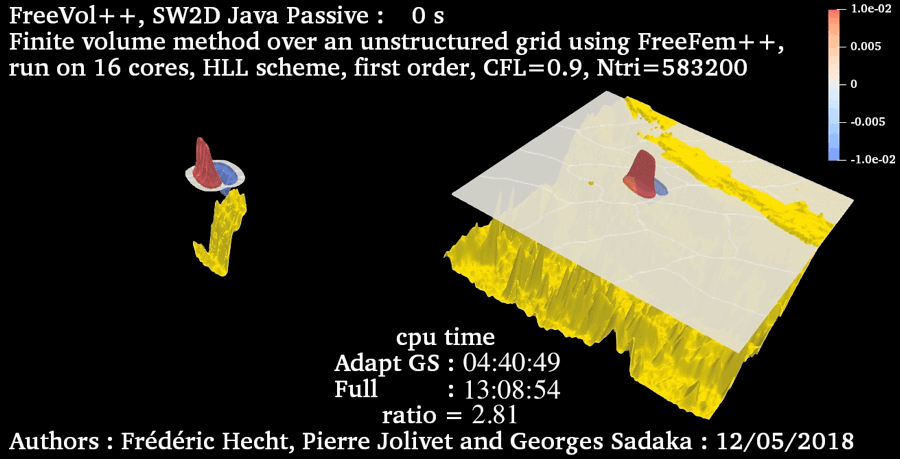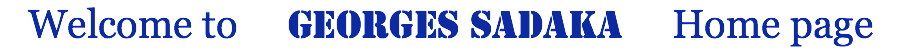Tsunami waves

Most Tsunami waves are generated from an underwater earthquake. We are interest to simulate the hole cycle of the Tsunami from generation till inundation. Till that end, we use here the simplified Boussinesq system of BBM type, derived by Mitsotakis, for generation and propagation of Tsunami waves, and for inundation we will use Shallow Water equations for instance, an other way to simulate the hole cycle of Tsunami will be presented later and which will take to account one system that will model the hole cycle of Tsunami. We apply a new domain adaptation technique defined by Sadaka.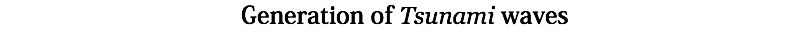The vertical displacement of the bottom during underwater earthquake near Java island - Indonesia in 17/07/2006. More details about the method used here can be found in Dutykh et al and Sadaka
Here, the dynamic bottom is define as :where H(t) is the Heaviside step function, T(t) is the scenario on the time dependence (instantaneous, linear, trigonometric, exponential, etc) and O(x,y) is the vertical component of the displacement vector computed from the Okada's formulas. First, we simulate the Okada's formulas in different positions :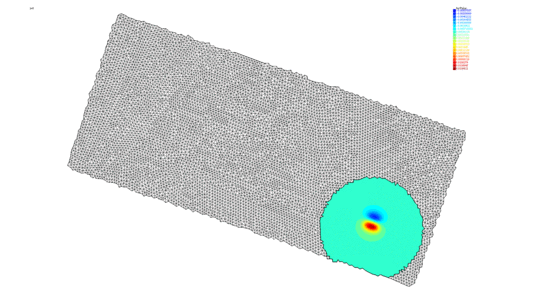then, we compute the vertical displacement of the bottom :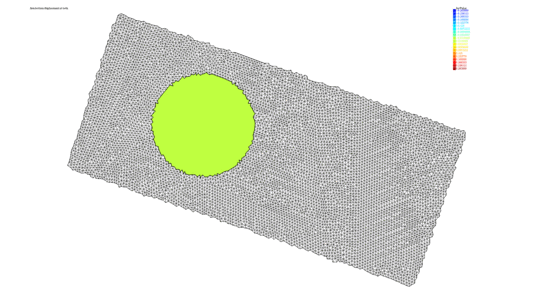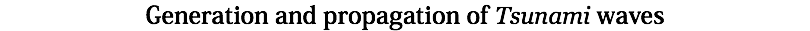For the generation and propagation of a Tsunami wave near Java island, we consider the simplified Boussinesq system of BBM type, derived by Mitsotakis, for more details see Sadaka: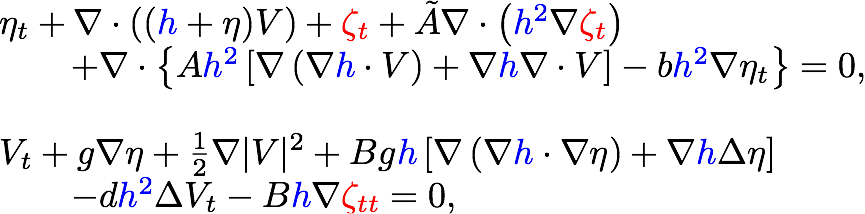we simulate here the generation and the propagation of the solution in a Passive case: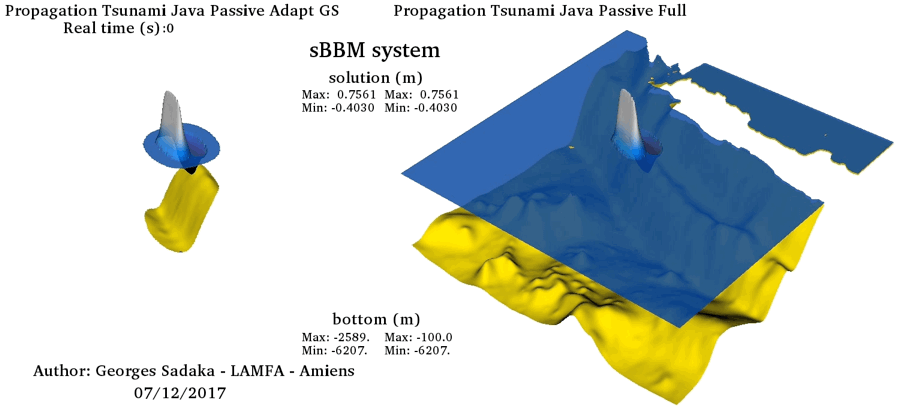and in the Active case: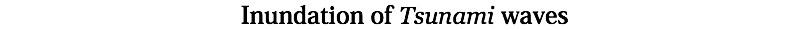The Shallow Water equations are routinely used to predict a Tsunami wave Run-up and, subsequently, constitute inundation maps for Tsunami hazard areas. We simulate here, the propagation of a wave that looks like a Tsunami generated by an earthquake, near Java island, using FreeVol++ with parallel mpi version and domain adaptation technique :